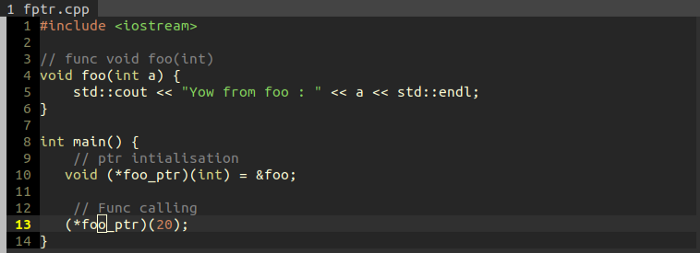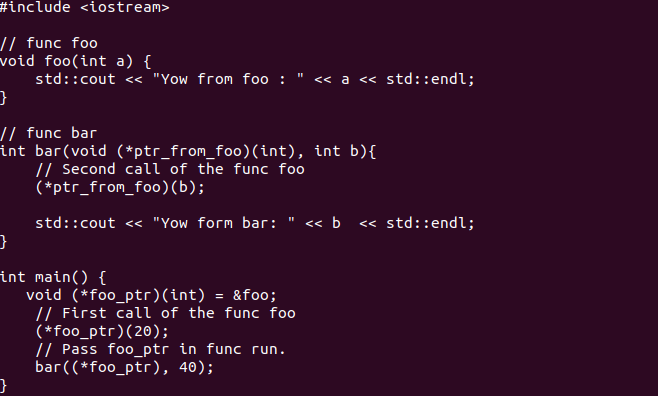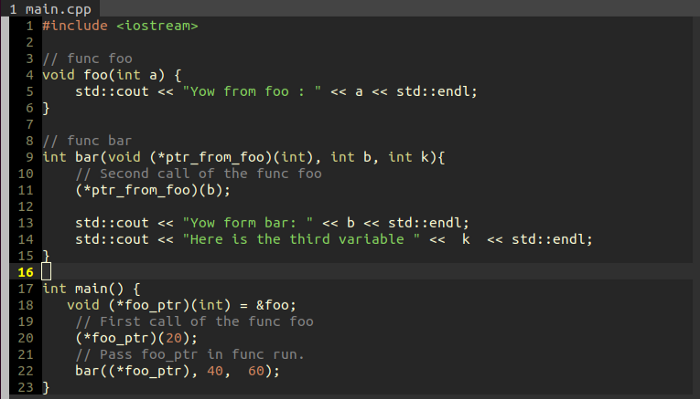RK Founder at HackersFriend Updated July 13, 2019, 12:27 p.m. ⋅ 1642 views

# Function Pointers in C++

As the title suggests this article contains two core concepts linked together which are FUNCTIONS and POINTERS.
So, let's start.

## What is FUNCTION POINTER?

A function pointer is a variable that holds the address of a function which can be called through that function pointer.
The utility of function pointers proves handy because of the feature of function that it encapsulates behavior which can be used for multiple use-cases which we will be discussing later.## FUNCTION POINTER SYNTAX:

You have a function called foo() which holds a specific memory location in the code segment.

``void foo(int);``

Now, this memory location needs to be held in a variable which is a pointer variable *ptr_foo like this:

``void (*ptr_foo)(int) = &foo;``

In the above command, we see void which denotes the return type of the function whose address is going to be held by the pointer which makes return type of pointer void too.

Next is the declaration syntax of a pointer and then pair of brackets holding a type which represents the argument data type that the function whose address the pointer holds.

On the right side of equals, we have &foo which explains that the address of function foo is being accessed and made to store in the variable ptr_foo using the “equals to” operator.

Key to writing the declaration for a function pointer is that just write the declaration of function but in place, fo func_name write *func_name.

## Reading a complex Function Pointer

Sometimes it gets confusing to read when there are many stars in a declaration.

``void * (*foo)(int *);``

Here, the key is to read inside-out that is the innermost element of the expression is, (*foo) which can be referred to as a function( foo) which returns a void* and takes an int * as an argument.

So *foo is the same as function foo (int *).

Now, as you have grasped the concept of function pointers and its syntax, let us see it in action in a use case where function pointer is made to pass as an argument in a function.

## Function as arguments to other functions

But before this, let us work on the reason for the need of function being passed in as an argument in another function.
Suppose we are working on a sort routine and want to let the user decide the process to achieve a sorted list, it could be ascending or descending.

We can go by flag-based approach and take flags as an indicator of the user’s choice but this is inflexible, allowing sort function a limited set of processing options.

So here we can adopt a nicer and clean way where we let the user pass in the function to the sort function by using the function pointer concept.
Here is a simple example of the same concept,In the above image, we see the function foo is being passed into the function bar by using function pointer concept that is foo_ptr.

Simple enough right?
But here comes an issue !!!

In the int main(), I use bar((*foo_ptr), 40) to call function bar but our prototype shows that function foo takes an int as an argument and function bar takes in a function pointer to foo and an int.

But when I call function bar I pass in only two arguments whereas I should pass three arguments, two for foo and one for bar.
This is packed under CLOSURE concept which functions lack and we will keep this concept for later articles.

So now in order to pass an argument for just function bar, I need to add one more variable in the argument and our code turns into this,Here we will get the third variable printed in line 14 with value 60.

This is one of the many use cases present for function pointers.

Explore more use cases and let me know in the comment for starters check out function callback using function pointers.

CHEERS !!!